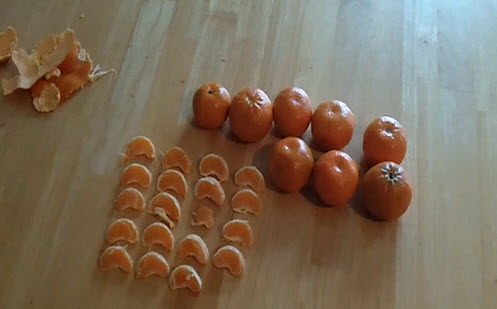Go to website

# Rows of oranges

In this Three-Act task, students are shown a video of someone peeling oranges and dividing them into segments. They then explore how many segments there will be when all the oranges have been peeled.

Year level(s) Year 1, Year 2, Year 3
Format Web page
Keywords rows, segments, total, addition, multiplication, array

## Curriculum alignment

Curriculum connections Numeracy
Strand and focus Number, Build understanding, Apply understanding
Topics Place value, Addition and subtraction, Multiplication and division, Patterns and algebra
AC: Mathematics (V9.0) content descriptions
AC9M2N05
Multiply and divide by one-digit numbers using repeated addition, equal grouping, arrays, and partitioning to support a variety of calculation strategies

AC9M1N04

Add and subtract numbers within 20, using physical and virtual materials, part-part-whole knowledge to 10 and a variety of calculation strategies

AC9M3N04
Multiply and divide one- and two-digit numbers, representing problems using number sentences, diagrams and arrays, and using a variety of calculation strategies

Numeracy progression Multiplicative strategies (P5)